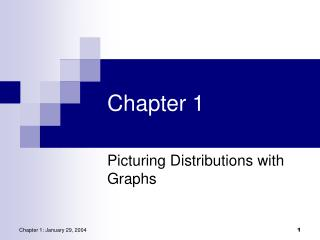DownloadDownload PresentationChapter 1

# Chapter 1

Télécharger la présentation## Chapter 1

- - - - - - - - - - - - - - - - - - - - - - - - - - - E N D - - - - - - - - - - - - - - - - - - - - - - - - - - -
##### Presentation Transcript

1. Chapter 1 Picturing Distributions with Graphs

2. Statistics Statistics is a science that involves the extraction of information from numerical data obtained during an experiment or from a sample. It involves: • the design of the experiment or sampling procedure, • the collection and analysis of the data, and • making inferences (statements, assumptions, guesses) about the population based upon information in a sample.

3. Individuals and Variables • Individuals • the objects described by a set of data • may be people, animals, or things • Variable • any characteristic of an individual • can take different values for different individuals

4. Variables • Categorical • Places an individual into one of several groups or categories • Quantitative (Numerical) • Takes numerical values for which arithmetic operations such as adding and averaging make sense

5. Example 1.1 • Individuals? • Variables? • Categorical? • Quantitative?

6. Distribution • Tells what values a variable takes and how often it takes these values • Can be a table, graph, or function

7. Displaying Distributions • Categorical variables • Pie charts • Bar graphs • Quantitative variables • Histograms • Stemplots (stem-and-leaf plots) • Time Plots

8. Example: Class Make-up on First Day Data Table

9. Example: Class Make-up on First Day Pie Chart

10. Example: Class Make-up on First Day Bar Graph

11. Example: U.S. Solid Waste (2000) Data Table

12. Example: U.S. Solid Waste (2000) Pie Chart

13. Example: U.S. Solid Waste (2000) Bar Graph

14. Example: U.S. Solid Waste (2000) Bar Graph

15. Histograms • For quantitative variables that take many values • Divide the possible values into class intervals (we will only consider equal widths) • Count how many observations fall in each interval (may change to percents) • Draw picture representing distribution

16. Case Study Weight Data Introductory Statistics classSpring, 1997 Virginia Commonwealth University

17. Weight Data

18. Weight Data: Frequency Table sqrt(53) = 7.2, or 8 intervals; range (260100=160) / 8 = 20 = class width

19. Number of students 100 120 140 160 180 200 220 240 260 280 Weight Data: Histogram Weight * Left endpoint is included in the group, right endpoint is not.

20. Histograms: Class Intervals • How many intervals? • One rule is to calculate the square root of the sample size, and round up. • Size of intervals? • Divide range of data (maxmin) by number of intervals desired, and round to convenient number • Pick intervals so each observation can only fall in exactly one interval (no overlap)

21. Examining the Distribution of Quantitative Data • Overall pattern of graph • Shape of the data • Center of the data • Spread of the data (Variation) • Deviations from overall pattern • Outliers

22. Shape of the Data • Symmetric • bell shaped • other symmetric shapes • Asymmetric • right skewed • left skewed • Unimodal, bimodal

23. SymmetricBell-Shaped SymmetricMound-Shaped SymmetricUniform

24. AsymmetricSkewed to the Left AsymmetricSkewed to the Right

25. Outliers • Extreme values that fall outside the overall pattern • May occur naturally • May occur due to error in recording • May occur due to error in measuring • Observational unit may be fundamentally different

26. Stemplots(Stem-and-Leaf Plots) • For quantitative variables • Separate each observation into a stem (first part of the number) and a leaf (the remaining part of the number) • Write the stems in a vertical column; draw a vertical line to the right of the stems • Write each leaf in the row to the right of its stem; order leaves if desired

27. Weight Data

28. 10 11 12 13 14 15 16 17 18 19 20 21 22 23 24 25 26 192 5 152 2 135 2 Weight Data:Stemplot(Stem & Leaf Plot) Key 20|3 means203 pounds Stems = 10’sLeaves = 1’s

29. 10 0166 11 009 12 0034578 13 00359 14 08 15 00257 16 555 17 000255 18 000055567 19 245 20 3 21 025 22 0 23 24 25 26 0 Weight Data:Stemplot(Stem & Leaf Plot) Key 20|3 means203 pounds Stems = 10’sLeaves = 1’s

30. Extended Stem-and-Leaf Plots If there are very few stems (when the data cover only a very small range of values), then we may want to create more stems by splitting the original stems.

31. 151516161717 Extended Stem-and-Leaf Plots Example: if all of the data values were between 150 and 179, then we may choose to use the following stems: Leaves 0-4 would go on each upper stem (first “15”), and leaves 5-9 would go on each lower stem (second “15”).

32. Time Plots • A time plot shows behavior over time. • Time is always on the horizontal axis, and the variable being measured is on the vertical axis. • Look for an overall pattern (trend), and deviations from this trend. Connecting the data points by lines may emphasize this trend. • Look for patterns that repeat at known regular intervals (seasonal variations).

33. Class Make-up on First Day(Fall Semesters: 1985-1993)

34. Average Tuition (Public vs. Private)